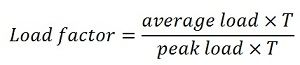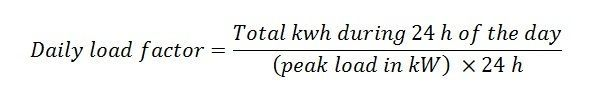Definition: Load factor is defined as the ratio of the average load over a given period to the maximum demand (peak load) occurring in that period. In other words, the load factor is the ratio of energy consumed in a given period of the times of hours to the peak load which has occurred during that particular period.Load factor means how efficiently we use energy. It is the measure of the utilisation of electrical energy during a given period to the maximum energy which would have been utilised in that period. The load factor plays an important role in the cost of generation per unit (kWh). The higher the load factor the smaller will be the generation cost for the same maximum demands. Load factor regarding energy,Depending on the number of hours in days, weeks, months, or years we define different load factors. For daily load factor, period T is taken as 24 hours; similarly, for weeks, months and years the different value of T is taken.

Mathematically,For calculating load factor, the following information is required;

1. Actual kilowatt hours used (kWh)
2. Peak kilowatt demand (kW)
3. Number of days

For Example:

Let total kWh = 36,0000 kWh
Demand = 100kW
The number of days = 30 days
Hours per day = 24 hours

SolutionSimilarly, we can calculate the annual, weeks and daily load factor. The value of the load factor is always less than 1 because the value of average load is always smaller than the maximum demand.

If the load factor is high (above 0.50), it shows that the power usage is relatively constant; if it is low, it means a high demand is set. For improving load factor, electrical load operating at the peak time of the day should be shifted to non-peaks time.

For example, let 12 machines are running at 10:00 am, instead of this it would be beneficial if 4 machines operating at 9:00 am, 5 machines operating at 10:00 am and 3 machines operating at 11:30 pm. So 12 machines are still running but not at the same time.

### 2 thoughts on “Load factor”

1. MY TOTAL CONNECTED LOAD IS 23560KVA (SINGLE PHASE), HOW CAN I CALCULATE DEMAND FACTOR,DIVERSITY FACTOR,LOAD FACTOR?